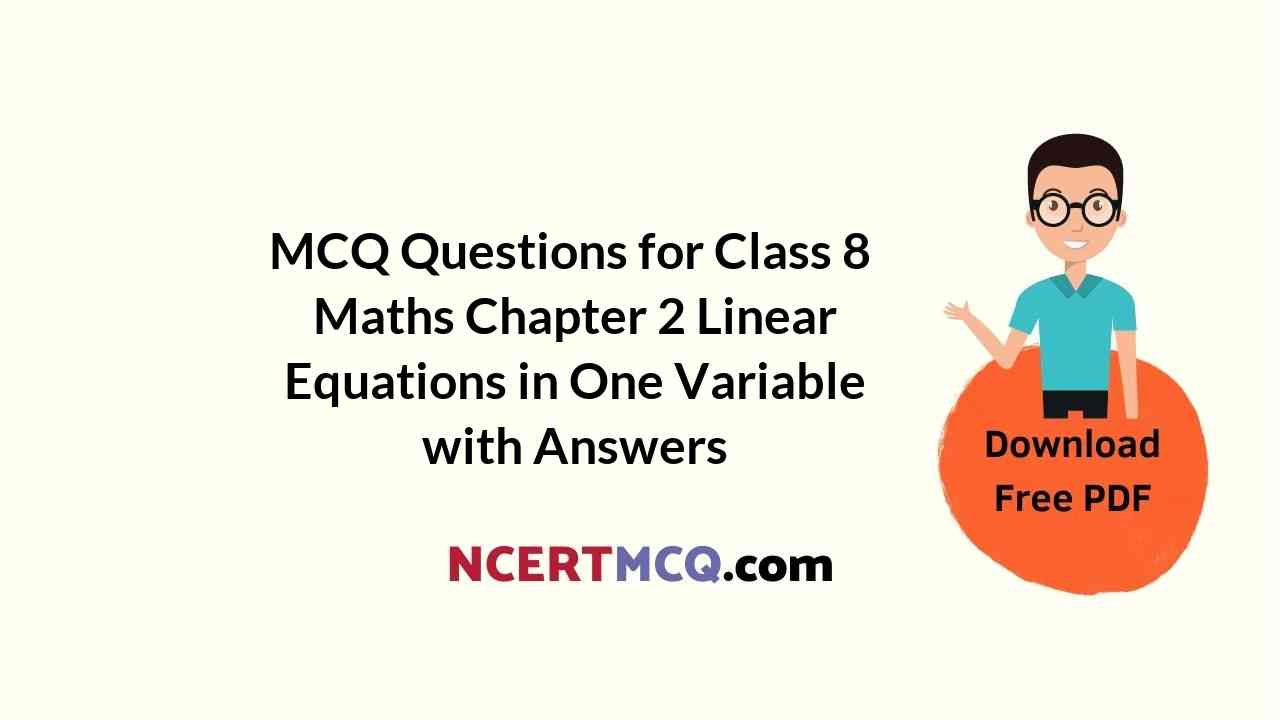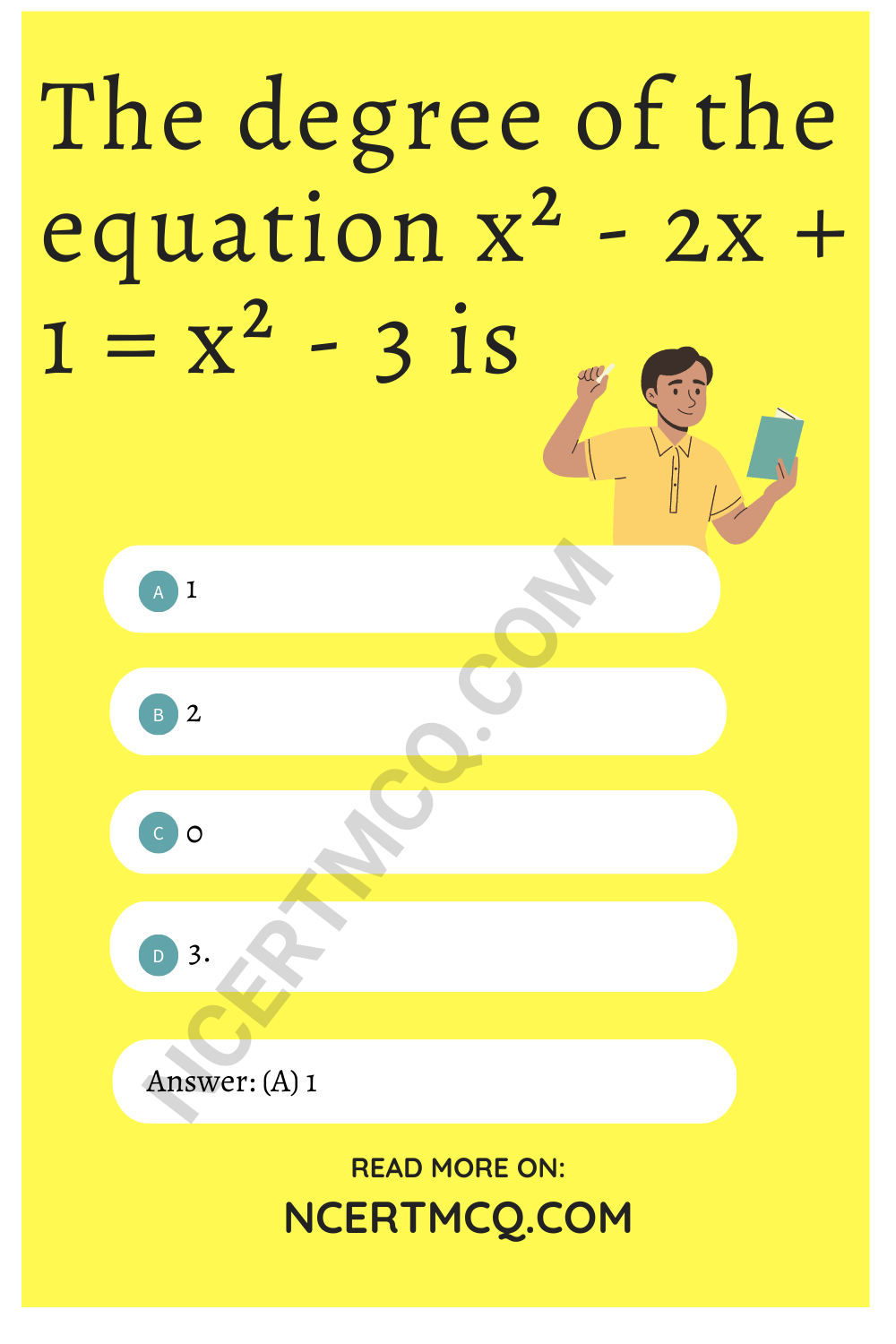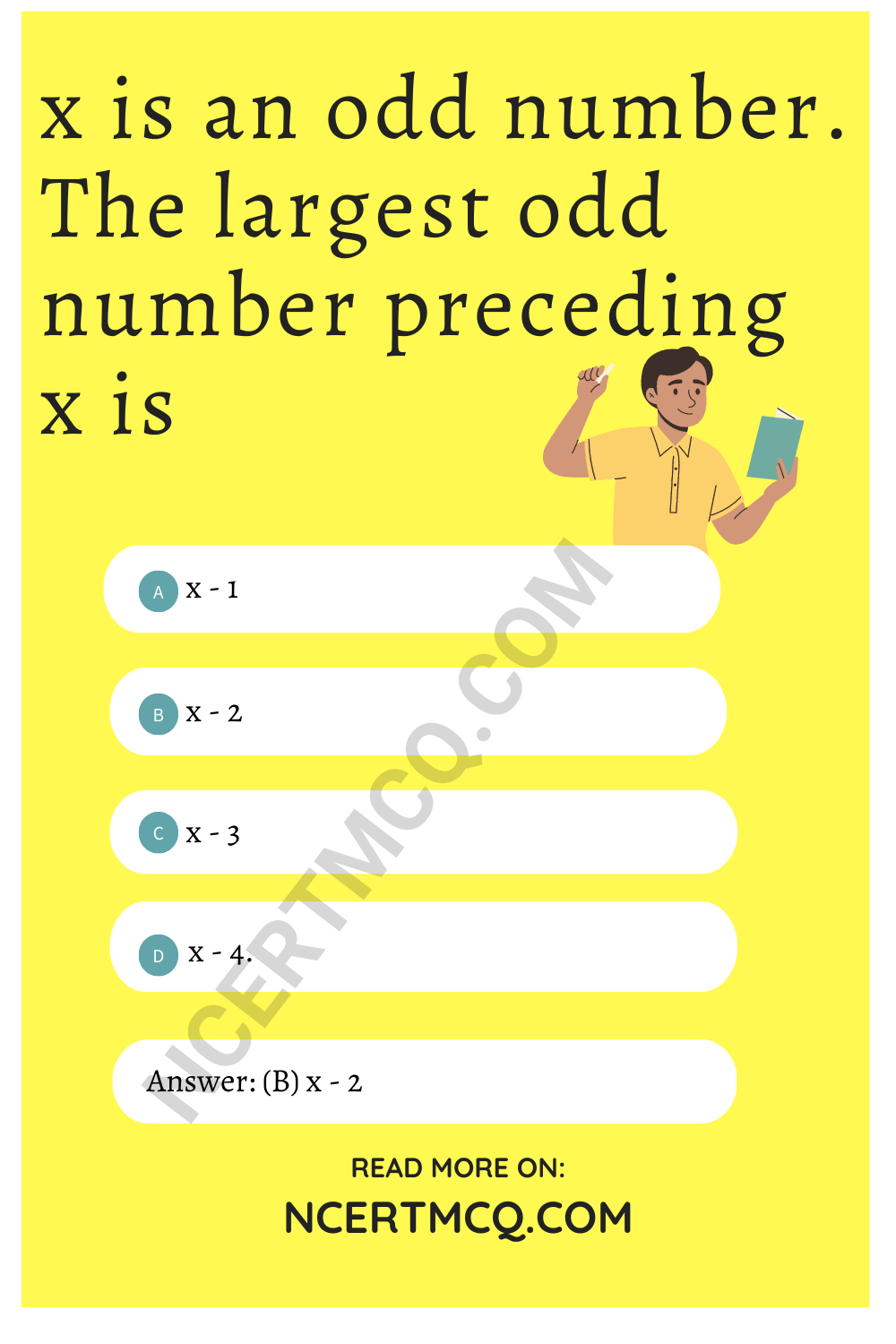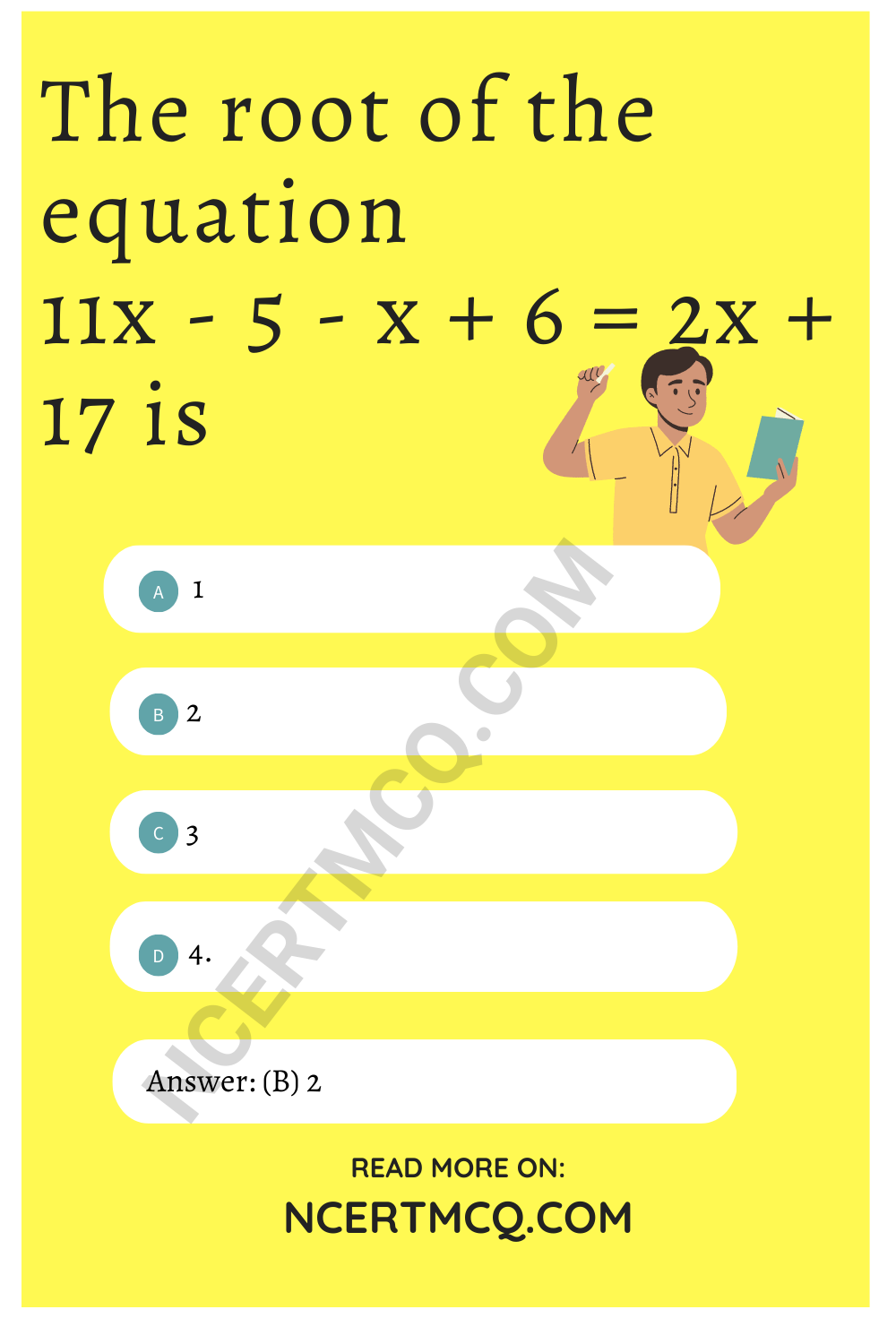Check the below Online Education NCERT MCQ Questions for Class 8 Maths Chapter 2 Linear Equations in One Variable with Answers Pdf free download. MCQ Questions for Class 8 Maths with Answers were prepared based on the latest exam pattern. We have provided Linear Equations in One Variable Class 8 Maths MCQs Questions with Answers to help students understand the concept very well. https://ncertmcq.com/mcq-questions-for-class-8-maths-with-answers/

Students can also refer to NCERT Solutions for Class 8 Maths Chapter 2 Linear Equations in One Variable for better exam preparation and score more marks.

## Linear Equations in One Variable Class 8 MCQs Questions with Answers

Class 8 Maths Chapter 2 MCQ Question 1.
The standard form of a linear equation in one variable x is
(a) ax + b = 0
(b) ax² + bx + c = 0
(c) ax³ + bx² + cx + d = 0
(d) ax4 + bx³ + cx² + dx + e = 0.

Linear Equations In One Variable Class 8 MCQ Question 2.
Of the following, the linear equation in one variable x, is
(a) $$\frac{4}{x}$$ = $$\frac{x}{4}$$
(b) $$\frac{1}{x}$$ + $$\frac{1}{x-1}$$ = 1
(c) $$\frac{x}{2}$$ + $$\frac{x}{3}$$ + $$\frac{1}{4}$$
(d) x² + 2x + 3 = 0.

MCQ Questions For Class 8 Maths Chapter 2 Question 3.
The degree of the equation x² – 2x + 1 = x² – 3 is
(a) 1
(b) 2
(c) 0
(d) 3.

Hint:
x² – 2x + 1 = x² – 3 ⇒ 2x = 4Class 8 Maths Ch 2 MCQ Question 4.
The statement ‘on adding 10 in a number, the number becomes 20’ in the form of an equation is
(a) x – 10 = 20
(b) x + 10 = 20
(c) 10x = 20
(d) $$\frac{x}{10}$$ = 20.

Hint:
Let the number be x.

MCQ Class 8 Maths Chapter 2 Question 5.
If 9 is added to a number, it becomes 25. This statement in the form of an equation is
(a) x + 9 = 25
(b) x – 9 = 25
(c) 9x = 25
(d) $$\frac{x}{9}$$ = 25.

Hint:
Let the number be x.

Class 8 Math Chapter 2 MCQ Question 6.
If 15 is subtracted from a number, it becomes -5. This statement in the form of an equation is
(a) x + 15 = -5
(b) x – 15 = 5
(c) x + 15 = 5
(d) x – 15 = -5.

Hint:
Let the number be x.

Linear Equations Class 8 MCQ Question 7.
Seven times a number is 42. This statement in the form of an equation is
(a) x + 7 = 42
(b) 7x = 42
(c) $$\frac{x}{7}$$ = 42
(d) x – 7 = 42.

Hint:
Let the number be x.

Linear Equations In One Variable MCQ Question 8.
A number when divided by 5 gives 6. This statement in the form of an equation is
(a) x – 5 = 6
(b) x + 5 = 6
(c) $$\frac{x}{5}$$ = 6
(d) 5x = 6.

Hint:
Let the number be x.

MCQ Questions For Class 8 Maths Ncert Chapter 2 Question 9.
A number when subtracted from 40 results into 15. This statement in the form of an equation is
(a) 40 – x = 15
(b) x – 40 = 15
(c) 40 + x = 15
(d) 40x = 15.

Hint:
Let the number be x.

Class 8 Chapter 2 Maths MCQ Question 10.
If 6 is added to 3 times of a number, it becomes 15. This statement in the form of an equation is
(a) 3x + 6 = 15
(b) 3x – 6 = 15
(c) 3x + 15 = 6
(d) $$\frac{3x}{6}$$ = 15.

Hint:
Let the number be x.

MCQ On Linear Equations For Class 8 Question 11.
On subtracting 30 from two times a number, we get 56. This statement in the form of an equation is
(a) 2x – 30 = 56
(b) 2x + 30 = 56
(c) 30 – 2x = 56
(d) $$\frac{30}{2x}$$ = 56.

Hint:
Let the number be x.

MCQ Questions For Class 8 Maths Chapter 2 With Answers Question 12.
The root of the equation z + 4 = -8 is
(a) 3
(b) -32
(c) 12
(d) 4.

Hint:
z + 4 = -8 ⇒ $$\frac{z}{4}$$ = -8
⇒ z = 4 (-8) = -32.

MCQ Questions For Class 8 Maths Linear Equations In One Variable Question 13.
The root of the equation 3x = $$\frac{20}{7}$$ – x is
(a) 10
(b) $$\frac{20}{21}$$
(c) –$$\frac{5}{7}$$
(d) $$\frac{5}{7}$$

Hint:
3x = $$\frac{20}{7}$$ – x ⇒ 3x + x = $$\frac{20}{7}$$
⇒ 4x = $$\frac{20}{7}$$ ⇒ x = $$\frac{20}{7×4}$$ = $$\frac{5}{7}$$

Maths Class 8 Chapter 2 MCQ Question 14.
The root of the equation 2x + 3 = 2(x – 4) is
(a) 2
(b) 4
(c) 0
(d) does not exist.

Hint:
2x + 3 = 2 (x – 4)
⇒ 2x + 3 = 2x – 8
⇒ 3 = -8 which is impossible.

Linear Equations In One Variable Class 8 MCQ With Answers Question 15.
The solution of the equation $$\frac{5}{x}$$ = 2 is
(a) 10
(b) $$\frac{2}{5}$$
(c) $$\frac{5}{2}$$
(d) $$\frac{1}{10}$$

Hint:
$$\frac{5}{x}$$ = 2
⇒ 2x = 5 ⇒ x = $$\frac{5}{2}$$

Question 16.
The largest number of the three consecutive numbers is x + 1. Then, the smallest number is
(a) x + 2
(b) x + 1
(c) x
(d) x – 1.

Hint:
x – 1, x, x + 1

Question 17.
If x is an even number then the consecutive even number is
(a) x + 1
(b) x + 2
(c) 2x
(d) x – 1.

Question 18.
x is an odd number. The largest odd number preceding x is
(a) x – 1
(b) x – 2
(c) x – 3
(d) x – 4.Question 19.
The difference of two numbers is 21. The larger number is x. The smaller number is
(a) 21 + x
(b) 21 – x
(c) x – 21
(d) -x – 21.

Hint:
x – smaller number = 21
⇒ smaller number = x – 21

Question 20.
In a two digit number, the unit’s digit is x and the ten’s digit is y. Then, the number is
(a) 10y + x
(b) 10x + y
(c) 10y – x
(d) 10x – y.

Hint:
Required number
= 10 × y + 1 × x = 10y + x.

Question 21.
When 9 is added to two times a number, we get 67. The number is
(a) 25
(b) 27
(c) 29
(d) 31.

Hint:
2x + 9 = 67 ⇒ x = 29.

Question 22.
The root of the equation –$$\frac{5}{4x}$$ = 15 is
(a) $$\frac{1}{12}$$
(b) –$$\frac{1}{12}$$
(c) $$\frac{1}{20}$$
(d) –$$\frac{1}{20}$$

Hint:
–$$\frac{5}{4x}$$ = 15 ⇒ x = –$$\frac{5}{4×15}$$ = –$$\frac{1}{12}$$

Question 23.
The value of x in $$\frac{3}{4}$$ x = 7 – x is
(a) 4
(b) 3
(c) $$\frac{7}{3}$$
(d) 7.

Hint:
$$\frac{3}{4}$$ x = 7 – x ⇒ $$\frac{3}{4}$$ x + x = 7
⇒ $$\frac{7}{4}$$ x = 7 ⇒ x = 4

Question 24.
$$\frac{3}{4}$$ part of a number is 5 more than its $$\frac{2}{3}$$ part. This statement in the form of an equation is
(a) $$\frac{2}{3}$$ x – $$\frac{3}{4}$$ x= 5
(5) $$\frac{2}{3}$$ x – 5 =$$\frac{3}{4}$$ x
(c) $$\frac{3}{4}$$ x = $$\frac{2}{3}$$ x + 5
(d) $$\frac{3}{4}$$ x – 5 = –$$\frac{2}{3}$$ x

Hint:
Let the number be x²

Question 25.
The value of x in –$$\frac{2}{3}$$ = 2x is 3
(a) $$\frac{1}{3}$$
(b) –$$\frac{1}{3}$$
(c) 3
(d) – 3.

Hint:
–$$\frac{2}{3}$$ = 2x ⇒ x = –$$\frac{1}{3}$$

Question 26.
The root of the equation 5x – 8 = 7 is
(a) 1
(b) 2
(c) 3
(d) -3.

Hint:
5x – 8 = 7 ⇒ 5x = 8 + 7 = 15
⇒ x = $$\frac{15}{5}$$ = 3.

Question 27.
The root of the equation x + 3 = 5 is
(a) 1
(b) 2
(0 -1
(d) -2.

Hint:
x + 3 = 5 ⇒ x = 5 – 3 = 2.

Question 28.
The root of the equation x – 8 = 2 is
(a) 2
(b) 8
(c) 6
(d) 10.

Hint:
x – 8 = 2 ⇒ x = 2 + 8 = 10.

Question 29.
The root of the equation 3x + 8 = 14 is
(a) 1
(b) 2
(c) -1
(d) $$\frac{1}{2}$$

Hint:
3x + 8 = 14 ⇒ 3x = 14 – 8 = 6
⇒ x = $$\frac{6}{3}$$ = 2.

Question 30.
The root of the equation 2y = 5(3 + y) is
(a) 5
(b) $$\frac{1}{5}$$
(c) -5
(d) –$$\frac{1}{5}$$

Hint:
2y = 5 (3 + y) ⇒ 2y = 15 + 5y
⇒ 5y – 2y = -15
⇒ 3y = -15 ⇒ y = –$$\frac{15}{3}$$ = -5

Question 31.
The root of the equation $$\frac{y}{3}$$ – 7 = 11 is
(a) 54
(b) -54
(c) 18
(d) -18

Hint:
$$\frac{y}{3}$$ – 7 = 11 ⇒ $$\frac{y}{3}$$ = 7 + 11 = 18
⇒ y = 3 × 18 = 54

Question 32.
The root of the equation 14 – x = 8 is
(a) 2
(b) 4
(c) 6
(d) 8.

Hint:
14 – x = 8 ⇒ x = 14 – 8 = 6

Question 33.
The root of the equation $$\frac{5x}{3}$$ = 30 is
(a) 9
(b) 12
(c) 15
(d) 18

Hint:
$$\frac{5x}{3}$$ = 30 ⇒ 5x = 3 × 30 = 90
⇒ x = $$\frac{90}{5}$$ = 18

Question 34.
The root of the equation 3y + 4 = 5y – 4 is
(a) 1
(b) 2
(c) 3
(d) 4.

Hint:
3y + 4 = 5y – 4 ⇒ 5y – 3y = 4 + 4
⇒ 2y = 8 ⇒ y = $$\frac{8}{2}$$ = 4

Question 35.
The root of the equation $$\frac{7x}{3}$$ = 3 is
(a) $$\frac{5x}{3}$$
(b) $$\frac{5x}{3}$$
(c) 3
(d) 7

Hint:
$$\frac{7}{x}$$ = 3 ⇒ 3x = 7 ⇒ x = $$\frac{7}{3}$$

Question 36.
The root of the equation 3x + 4 = 13 is
(a) 1
(b) 2
(c) 3
(d) 4.

Hint:
3x + 4 = 13 ⇒ 3x = 13 – 4 = 9
⇒ x = $$\frac{9}{3}$$ = 3

Question 37.
The root of the equation 9z – 15 = 9 – 3z is
(a) 1
(b) 2
(c) 3
(d) 4.

Hint:
9z – 15 = 9 – 3z ⇒ 9z + 3z = 9 + 15
⇒ 12x = 24 ⇒ z = $$\frac{24}{12}$$ = 2

Question 38.
The root of the equation $$\frac{4x}{7}$$ – 12 = 0 is
(a) 7
(b) 14
(c) 21
(d) -21

Hint:
$$\frac{4x}{7}$$ – 12 = 0 ⇒ $$\frac{4x}{7}$$ = 12
⇒ 4x = 7 × 12 = 84
⇒ x = $$\frac{84}{4}$$ = 21

Question 39.
The root of the equation 3x = $$\frac{20}{7}$$ – x is
(a) $$\frac{7}{5}$$
(b) $$\frac{5}{7}$$
(c) –$$\frac{7}{5}$$
(d) –$$\frac{5}{7}$$

Hint:
3x = $$\frac{20}{7}$$ – x ⇒ 3x + x = $$\frac{20}{7}$$
⇒ 4x = $$\frac{20}{7}$$ ⇒ x = $$\frac{20}{7×4}$$ = $$\frac{5}{7}$$

Question 40.
The root of the equation 2y = 5 (7 – y ) is
(a) 5
(b) -5
(c) 3
(d) -3.

Hint:
2y = 5(7 – y) ⇒ 2y = 35 – 5y
⇒ 2y + 5y = 35
⇒ 7y = 35 ⇒ y = $$\frac{35}{7}$$ = 5

Question 41.
The root of the equation
(2x – 1) + (x – 1) = x + 2 is
(a) 1
(b) 2
(c) -1
(d) -2.

Hint:
(2x – 1) + (x – 1) = x + 2
⇒ 2x = 4 ⇒ x = $$\frac{4}{2}$$ = 2.

Question 42.
The root of the equation
13x – 14 = 9x + 10 is
(a) 1
(b) 2
(c) 3
(d) 6.

Hint:
13x – 14 = 9x + 10 ⇒ 13x – 9x = 10 + 14
⇒ 4x = 24 ⇒ x = $$\frac{24}{4}$$ = 6

Question 43.
The root of the equation
11x – 5 – x + 6 = 2x + 17 is
(a) 1
(b) 2
(c) 3
(d) 4.

Hint:
11x – 5 – x + 6 = 2x + 17
⇒ 8x = 16 ⇒ x = 2.Question 44.
The root of the equation $$\frac{2}{3}$$ y = $$\frac{5}{12}$$ is
(a) $$\frac{8}{5}$$
(b) $$\frac{5}{8}$$
(c) 5
(d) 8

Hint:
$$\frac{2}{3}$$ y = $$\frac{5}{12}$$
⇒ y = $$\frac{5}{12}$$ × $$\frac{3}{2}$$ = $$\frac{5}{8}$$

Question 45.
The root of the equation 7 (x – 1) = 21 is
(a) 1
(b) 2
(c) 3
(d) 4.

Hint:
7 (x – 1) = 21 ⇒ x – 1 = $$\frac{21}{7}$$ = 3
⇒ x = 4.

Question 46.
The root of the equation $$\frac{3}{2}$$ x = – 27 is
(a) 6
(b) 12
(c) 18
(d) -18

Hint:
$$\frac{5}{12}$$ x = -27 ⇒ x = –$$\frac{27×2}{3}$$ = -18.

Question 47.
If two angles are complementary and one angle is 10° greater than the other, then the smaller angle of the two is
(a) 40°
(b) 50°
(c) 90°
(d) 180°.

Hint:
x° = (90° – x°) + 10°
⇒ 2x° = 100° ⇒ x° = 50°
⇒ 90° – x° = 40°.

Question 48.
If two angles are supplementary and one angle is double the other, then the larger angle is
(a) 60°
(b) 90°
(c) 120°
(d) 180°.

Hint:
x° + 2x° = 180° ⇒ 3x° = 180°
⇒ x° = 60° ⇒ 2x° = 120°

Question 49.
Twice a number is as much greater than 30 as the three times of the number less than 60. The number is
(a) 6
(b) 9
(c) 12
(d) 18.

Hint:
2x – 30 = 60 – 3x ⇒ 5x = 90
⇒ x = 18.

Question 50.
One number is greater than the other number by 3. The sum of two numbers is 23. The two numbers are
(a) 13, 10
(b) 14, 9
(c) 12, 11
(d) 15, 8.